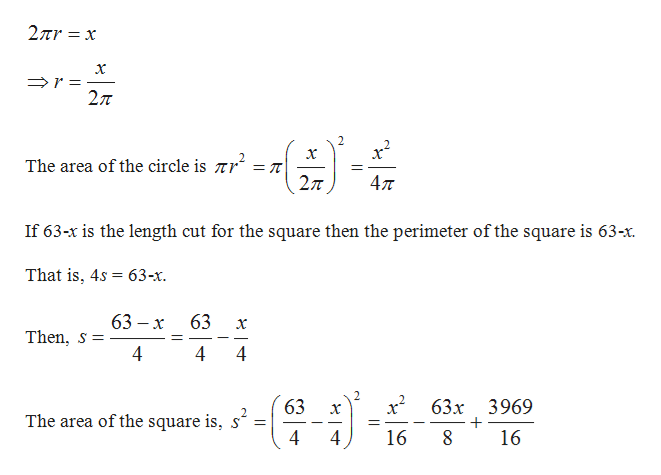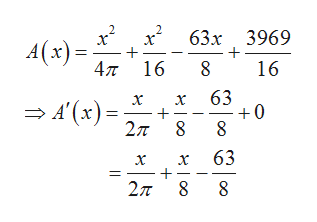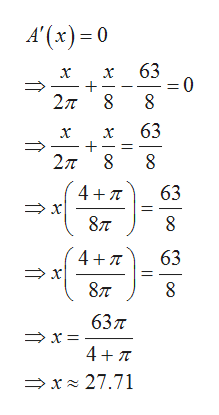# A piece of wire of length 63 is cut, and the resulting tow pieces are formed to make a circle and a square. Where should the wire be cut to (a) minimize and (b) maximize the combined area of the circle and the square?(a) Let x be the amount of wire used for the circle. What is the function A, the combined ara of the circle and square in terms of x?A= ______ (type an expression, type the exact number using pi as needed)To minimize the combined area, the wire should be cut so that a length of ____ is used for the circle and a length of ____ is used for the square. (round to nearest thousandth)(b) To maximize the combined area, the wire should be cut so that a length of ____ is used for the circle and a length of ____ is used for the square. (round to nearest thousandth)

Question

A piece of wire of length 63 is cut, and the resulting tow pieces are formed to make a circle and a square. Where should the wire be cut to (a) minimize and (b) maximize the combined area of the circle and the square?

(a) Let x be the amount of wire used for the circle. What is the function A, the combined ara of the circle and square in terms of x?

A= ______ (type an expression, type the exact number using pi as needed)

To minimize the combined area, the wire should be cut so that a length of ____ is used for the circle and a length of ____ is used for the square. (round to nearest thousandth)

(b) To maximize the combined area, the wire should be cut so that a length of ____ is used for the circle and a length of ____ is used for the square. (round to nearest thousandth)

check_circleExpert Solution
Step 1

The total length of the wire is 63.

Let x be the length of the wire cut for the circle.

Then, 63-x is the length of the ire cut for the square.

If x is the length cut for the circle then the circumference of the circle is x.

That is,help_outlineImage Transcriptionclose2πr x 27T - x The area of the circle is Tr 27T 47T If 63-x is the length cut for the square then the perimeter of the square is 63-x. That is, 4s 63-x 63 — х 63 Then, s 4 4 4 2 63 63x 3969 x The area of the square is, s' 4 16 fullscreen
Step 2

Total area of the circle and the square is,help_outlineImage Transcriptioncloseх* 63х 3969 A(x) 4л 16 8 16 63 +0 х х — 4 (к) -, 2л 8 63 х х 2л о о fullscreen
Step 3

Compute the maximum or mi...help_outlineImage TranscriptioncloseA'(x)0 63 x 0 = 2π 8 8 63 2π8 47T 63 877 8 4 T 63 x 877 637 x 4 TT x 27.71 fullscreen

### Want to see the full answer?

See Solution

#### Want to see this answer and more?

Solutions are written by subject experts who are available 24/7. Questions are typically answered within 1 hour*

See Solution
*Response times may vary by subject and question
Tagged in

### Other